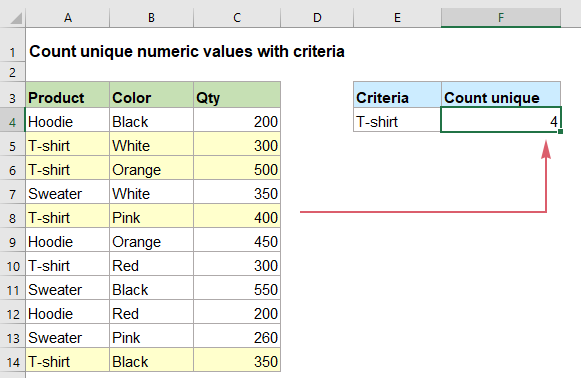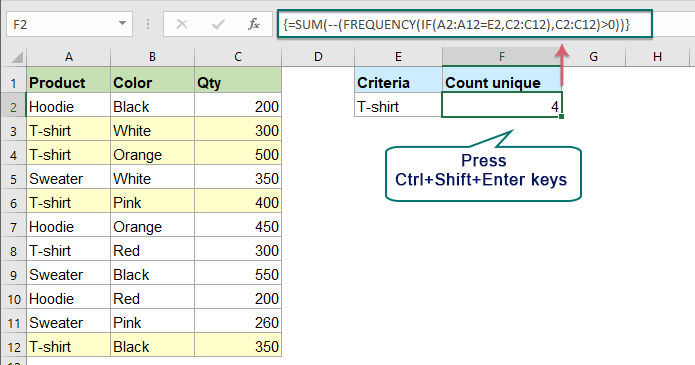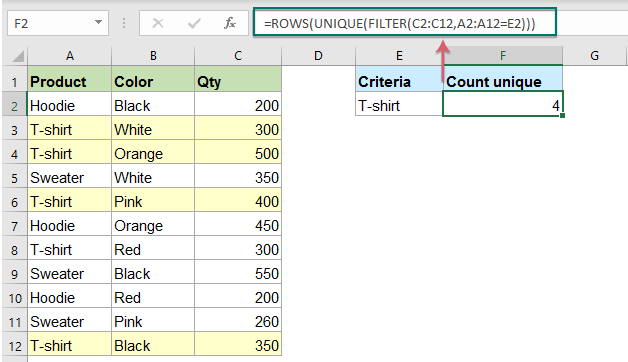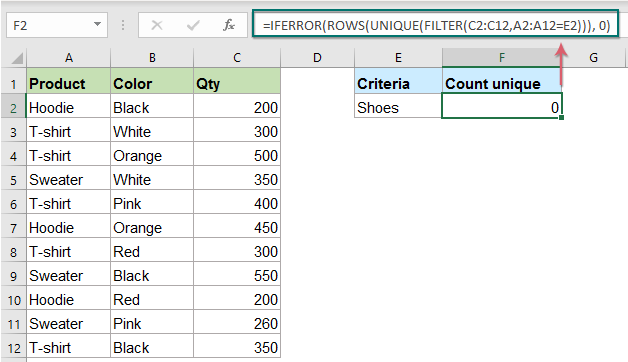## Count unique numeric values based on criteria in Excel

In Excel worksheet, you may suffer from a problem that count the number of unique numeric values based on a specific condition. For example, how can I count the unique Qty values of the product “T-shirt” from the report as below screenshot shown? In this article, I will show some formulas to achieve this task in Excel.#### Count unique numeric values based on criteria in Excel 2019, 2016 and earlier

In Excel 2019 and earlier versions, you can combine the SUM, FREQUENCY and IF functions to create a formula for counting unique values based on criteria, the generic syntax is:

{=SUM(--(FREQUENCY(IF(criteria_range=criteria,range),range)>0))}
Array formula, should press Ctrl + Shift + Enter keys together.
• criteria_range: The range of cells to match the criteria you specified;
• criteria: The condition that you want to count unique values based on;
• range: The range of the cells with unique values to be counted.

Please apply the below formula into a blank cell, and press Ctrl + Shift + Enter keys to get the correct result, see screenshot:

=SUM(--(FREQUENCY(IF(A2:A12=E2,C2:C12),C2:C12)>0))##### Explanation of the formula:

=SUM(--(FREQUENCY(IF(A2:A12=E2,C2:C12),C2:C12)>0))

• IF(A2:A12=E2,C2:C12): This IF function returns the value in column C if the product in column A is “T-shirt”, the result is an array like this: {FALSE;300;500;FALSE;400;FALSE;300;FALSE;FALSE;FALSE;350}.
• FREQUENCY(IF(A2:A12=E2,C2:C12),C2:C12)= FREQUENCY({FALSE;300;500;FALSE;400;FALSE;300;FALSE;FALSE;FALSE;350},{200;300;500;350;400;450;300;550;200;260;350}): The FREQUENCE function is used to count each of the numeric values in the array list, and return the result as this: {0;2;1;1;1;0;0;0;0;0;0;0}.
• --(FREQUENCY(IF(A2:A12=E2,C2:C12),C2:C12)>0)=--({0;2;1;1;1;0;0;0;0;0;0;0}>0): Test if each value in the array is greater than 0, and get the result as this: {FALSE;TRUE;TRUE;TRUE;TRUE;FALSE;FALSE;FALSE;FALSE;FALSE;FALSE;FALSE}. And then, the double negative sign converts the TRUEs and FALSEs into 1s and 0s, returning an array like this: {0;1;1;1;1;0;0;0;0;0;0;0}.
• SUM(--(FREQUENCY(IF(A2:A12=E2,C2:C12),C2:C12)>0))=SUM({0;1;1;1;1;0;0;0;0;0;0;0}): Finally, use the SUM function to add these values up, and get the total number: 4.

Tips:

If you want to count the unique values based on more than one condition, you just need to add other criteria to the formula with * character:

=SUM(--(FREQUENCY(IF((criteria,_range1=criteria1)* (criteria,_range2=criteria2)*…,range),range)>0))

#### Count unique numeric values based on criteria in Excel 365

In Excel 365, the combination of the ROWS, UNIQUE and FILTER functions can help to count unique numeric values based on criteria, the generic syntax is:

=ROWS(UNIQUE(FILTER(range,criteria_range=criteria)))
• range: The range of the cells with unique values to be counted.
• criteria_range: The range of cells to match the criteria you specified;
• criteria: The condition that you want to count unique values based on;

Please copy or enter the following formula into a cell, and press Enter key to return the result, see screenshot:

=ROWS(UNIQUE(FILTER(C2:C12,A2:A12=E2)))##### Explanation of the formula:

=ROWS(UNIQUE(FILTER(C2:C12,A2:A12=E2)))

• A2:A12=E2: This expression checks if the value in cell E2 exists in the range A2:A12, and get this result: {FALSE;TRUE;TRUE;FALSE;TRUE;FALSE;TRUE;FALSE;FALSE;FALSE;TRUE}.
• FILTER(C2:C12,A2:A12=E2): The FREQUENCE function is used to count each of the numeric values in the array list, and return the result as this: {0;2;1;1;1;0;0;0;0;0;0;0}.
• UNIQUE(FILTER(C2:C12,A2:A12=E2))=UNIQUE({300;500;400;300;350}): Here, the UNIQUE function is used to extract unique values from the list array to get this result: {300;500;400;350}.
• ROWS(UNIQUE(FILTER(C2:C12,A2:A12=E2)))=ROWS({300;500;400;350}): The ROWS function returns the number of rows based on a cell range or array, so, the result is: 4.

Tips:

1. If the matched value does not exist in the data range, you will get an error value, to replace the error value with 0, please apply the following formula:

=IFERROR(ROWS(UNIQUE(FILTER(C2:C12,A2:A12=E2))), 0)2. To count the unique values based on more than one condition, you just need to add other criteria to the formula with * character like this:

=ROWS(UNIQUE(FILTER(range,(criteria_range1=criteria1)* (criteria_range2=criteria2)*…)))

#### Relative function used:

• SUM:
• The Excel SUM function returns the sum of values supplied.
• FREQUENCY:
• The FREQUENCY function calculates how often values occur within a range of values, and then returns a vertical array of numbers.
• ROWS:
• The ROWS function returns the number of rows in a given reference or array.
• UNIQUE:
• The UNIQUE function returns a list of unique values in a list or range.
• FILTER:
• The FILTER function helps to filter a range of data based on criteria you define.

#### More articles:

• Count Unique Numeric Values Or Dates In A Column
• Supposing you have a list of numbers which contain some duplicates, now, you want to count the number of the unique values or the values appear only once in the list as below screenshot shown. In this article, we will talk about some useful formulas for solving this task in Excel quickly and easily.
• Count All Matches / Duplicates Between Two Columns
• Comparing two columns of data and count all matches or duplicates in the two columns may be a common task for most of us. For example, you have two columns of names, some names appear both in the first and second columns, now, you want to count all matched names (the matches located anywhere within the two columns) between two columns as below screenshot shown, this tutorial will introduce some formulas for achieving this goal in Excel.
• Count Number Of Cells Equals To One Of Many Values
• Supposing, I have a list of products in column A, now, I want to get the total number of specific products Apple, Grape and Lemon which listed in range C4:C6 from column A as below screenshot shown. Normally, in Excel, the simple COUNTIF and COUNTIFS functions will not work in this scenario. This article, I will talk about how to solve this job quickly and easily with the combination of SUMPRODUCT and COUNTIF functions.

### The Best Office Productivity Tools

#### Kutools for Excel - Helps You To Stand Out From Crowd

 Popular Features: Find, Highlight or Identify Duplicates  |  Delete Blank Rows  |  Combine Columns or Cells without Losing Data  |  Round without Formula ... Super VLookup: Multiple Criteria  |  Multiple Value  |  Across Multi-Sheets  |  Fuzzy Lookup... Adv. Drop-down List: Easy Drop Down List  |  Dependent Drop Down List  |  Multi-select Drop Down List... Column Manager: Add a Specific Number of Columns  |  Move Columns  |  Toggle Visibility Status of Hidden Columns  |  Compare Columns to Select Same & Different Cells ... Featured Features: Grid Focus  |  Design View  |  Big Formula Bar  |  Workbook & Sheet Manager | Resource Library (Auto Text)  |  Date Picker  |  Combine Worksheets  |  Encrypt/Decrypt Cells  |  Send Emails by List  |  Super Filter  |  Special Filter (filter bold/italic/strikethrough...) ... Top 15 Toolsets:  12 Text Tools (Add Text, Remove Characters ...)  |  50+ Chart Types (Gantt Chart ...)  |  40+ Practical Formulas (Calculate age based on birthday ...)  |  19 Insertion Tools (Insert QR Code, Insert Picture from Path ...)  |  12 Conversion Tools (Numbers to Words, Currency Conversion ...)  |  7 Merge & Split Tools (Advanced Combine Rows, Split Excel Cells ...)  |  ... and more

Kutools for Excel Boasts Over 300 Features, Ensuring That What You Need is Just A Click Away...#### Office Tab - Enable Tabbed Reading and Editing in Microsoft Office (include Excel)

• One second to switch between dozens of open documents!
• Reduce hundreds of mouse clicks for you every day, say goodbye to mouse hand.
• Increases your productivity by 50% when viewing and editing multiple documents.
• Brings Efficient Tabs to Office (include Excel), Just Like Chrome, Edge and Firefox.